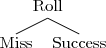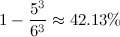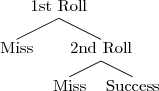Page 1 of 1

### Axis & Allies dice probability

Posted: Mon Jul 22, 2013 8:42 am UTC
I've been thinking about probability in Axis & Allies dice rolls, where a single d6 roll is used to determine a successful hit or miss (such as a torpedo attack in Naval Miniatures, where 1-5 count as a miss and a 6 counts as a successful hit.)I know based on reading this, that for a given number of rolls, the probability of a hit on the above outcome tree can be calculated with the formula:M = Number of possible die outcomes resulting in a miss
T = Total number of possible die outcomes
n = Number of dice rolled

So for example, if a submarine performs a torpedo attack that calls for 3 dice rolls, the chance of at least one successful hit would be:I'm trying to figure out a formula for calculating the probability of at least one successful hit if a success were to require a second die roll:At the moment, I'm lost as to how to fit that second roll into the probability equation. Any help would be greatly appreciated.

### Re: Axis & Allies dice probability

Posted: Mon Jul 22, 2013 8:51 am UTC
I'm not sure I understand the question.

You roll three times and want to know what are the chances you get at least one 6?
Exactly the same as before.

The second roll doesn't matter because you've already hit. Your trial is already a success; you have "at least one 6".

### Re: Axis & Allies dice probability

Posted: Mon Jul 22, 2013 8:54 am UTC
No, I'm trying to figure out the probability where success is determined by two consecutive rolls instead of a single roll. For example, someone has to roll a 4, 5, or 6 followed by a 6 in order to have a success.

### Re: Axis & Allies dice probability

Posted: Mon Jul 22, 2013 9:05 am UTC
Your first post doesn't state that at all...

So success is given by a roll of 4, 5 or 6 followed by a 6? All other rolls are failure?

Roll 1 has probability 1/2.
Roll 2 has probability 1/6.
All other outcomes are failures, so it's a simple multiplication from here.

### Re: Axis & Allies dice probability

Posted: Mon Jul 22, 2013 3:21 pm UTC
musashi1600 wrote:I know based on reading this, that for a given number of rolls, the probability of a hit on the above outcome tree can be calculated with the formula:A better formula is that the probability of at least 1 success in n rolls, where each roll has a probability p of success, is 1-(1-p)n. This is because each roll has a probability (1-p) of failure, so the probability than in n rolls all will fail is (1-p)n. That formula is the same as the one you gave in case there are M ways to fail out of T equally probable alternatives, but is better because it applies in cases where the single-trial success probability p is computed some other way.

Now, if your success requires two rolls, you can still easily work out the probability of success. For example, if you need a 4+ followed by a 6 on two dice, there's a 3/6 * 1/6 = 1/12 chance of a success. So this formula gives 1-(1-1/12)n = 1-(11/12)n probability for at least 1 success in n attempts.

### Re: Axis & Allies dice probability

Posted: Fri Jul 26, 2013 11:26 pm UTC
Yes, that's exactly what I'm looking for. Thanks!

### Re: Axis & Allies dice probability

Posted: Mon Oct 20, 2014 2:07 am UTC
@ skeptical scientist:

Hi! Another non-mathematician interested in calculating probability for a specific case. Might I ask you a quick one related to this?

So the elegant solution you gave to find die roll probabilities for at least one 'hit' is 1- (1-p)2; however, I'm trying to find a solution for:

Rolling 4 ten-sided dice, what is the probability of rolling a 1, 2 or 3 for at least two of those dice? I'm trying to work with and adapt the equation you gave above but I feel like a Kerbal.

Best,

Sobriquet1

### Re: Axis & Allies dice probability

Posted: Fri Oct 24, 2014 11:08 pm UTC
The chance of rolling 1,2, or 3 with no dice is .7^4

The chance of rolling 1,2, or 3 with exactly one die is (.3)(.7^3)*4

So the chance of rolling with at least two dice is 1 - .7^4 - (.3)(.7^3)*4 = .3483

### Re: Axis & Allies dice probability

Posted: Sat Oct 25, 2014 5:39 pm UTC
A while back I played a flash game where you had units of different strengths from 1 through 6. If you had a strength of 1, you got 1 dice, a strength of 2 you had 2 dice, etc. You win if the sum of your dice is greater than the sum of your opponent's dice. I wrote a program to calculate the odds of winning, which I might be able to dig up somewhere, but I'm really not sure if I still have it. It was almost a brute force, but I iterated through the distinct rolls and then calculated the number of permutations (IIRC). Reading this thread, I'm curious, is there a mathematical formula to calculate if you roll m dice and your opponent rolls n dice, what is the probability that you will win, tie, or lose?

### Re: Axis & Allies dice probability

Posted: Tue Oct 28, 2014 8:30 pm UTC
musashi1600 wrote:No, I'm trying to figure out the probability where success is determined by two consecutive rolls instead of a single roll. For example, someone has to roll a 4, 5, or 6 followed by a 6 in order to have a success.

That's...1/2 for the first roll, 1/6 for the second. Since both need to be true, it's a straight multiplication, resulting in 1/12.

If you want crazy dice probability, I suggest checking out unusual dice rules like "exploding" dice, where rolling certain results add dice to the pool, etc. The math on that gets to be a little more fun.

Thesh wrote:A while back I played a flash game where you had units of different strengths from 1 through 6. If you had a strength of 1, you got 1 dice, a strength of 2 you had 2 dice, etc. You win if the sum of your dice is greater than the sum of your opponent's dice. I wrote a program to calculate the odds of winning, which I might be able to dig up somewhere, but I'm really not sure if I still have it. It was almost a brute force, but I iterated through the distinct rolls and then calculated the number of permutations (IIRC). Reading this thread, I'm curious, is there a mathematical formula to calculate if you roll m dice and your opponent rolls n dice, what is the probability that you will win, tie, or lose?

Sure. And for most common permutations(ie, batch of d6s), there's a calculator out there somewhere. I used to be somewhat into this back when I played warhammer.

I'm feeling to lazy to attempt to fully generalize it at the moment, but for standard addition, you're going to see ever increasing quantities of dice more closely approach a normal distribution centered on the average. With "bucket of dice" levels thrown, luck really stops playing a large part in it, and ties of course become insignificant.